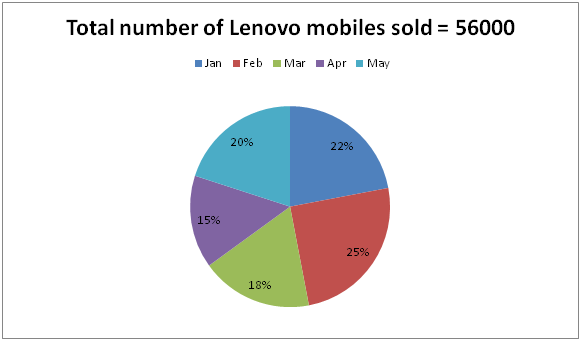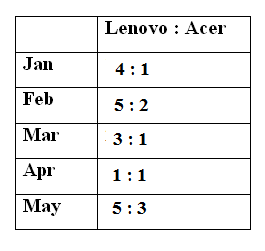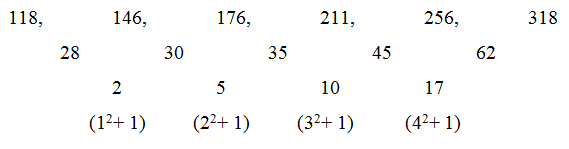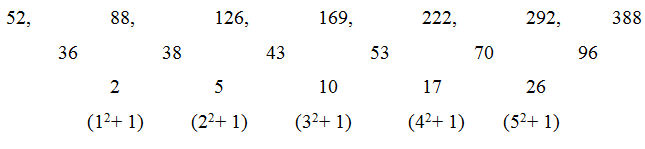# SBI PO Prelims Quantitative Aptitude Questions 2019 – Day 15

SBI PO 2019 Notification will be expected soon. It is one of the most expected recruitment among the banking aspirants. Every year the exam pattern for SBI PO has been changing. Depends upon the changing of exam pattern the questions are quite harder compare to the previous year. So the questions are in high level than the candidate’s assumption.

As per the latest trend, our IBPS Guide is providing the updated New Exam Pattern Quantitative Aptitude questions for SBI PO 2019 Day 14. Our Skilled experts were mounting the questions based on the aspirant’s needs. So candidates shall start your preparation and practice on daily basis with our SBI PO pattern quantitative aptitude questions 2019 day 14. Start your effective preparation from the right beginning to get success in upcoming SBI PO 2019.

[WpProQuiz 5248]

### Click Here for SBI PO Pre 2019 High-Quality Mocks Exactly on SBI Standard

Directions (1 – 5): The questions below are based on the given Series-I. The series-I satisfy a certain pattern, follow the same pattern in Series-II and answer the questions given below.

1) I) 12, 16, 48, 180, 784

II) 5 ………. 616. If 616 is nth term, then what value should come in place of (n – 2)th term?

a) 56

b) 72

c) 68

d) 34

e) 30

2) I) 117, 122, 147, 272, 897, 4022

II) 21 ……… 801. If 801 is nth term, then what value should come in place of (n + 1)th term?

a) 3877

b) 3926

c) 3729

d) 3652

e) None of these

3) I) 8, 5, 7, 18, 80, 656

II) 14 ……… 848. If 848 is nth term, then find the value of n?

a) 8

b) 7

c) 6

d) 5

e) None of these

4) I) 118, 146, 176, 211, 256, 318

II) 52, 88 …….. 388. If 388 is nth term, then find the value of n?

a) 7

b) 6

c) 5

d) 8

e) None of these

5) I) 7, 17, 37, 77, 157, 317

II) 21 ……. 381. If 381 is nth term, then find the value of (n + 2)th term?

a) 1346

b) 1602

c) 1533

d) 765

e) 1428

Directions (Q. 6 – 10) Study the following information carefully and answer the given questions:

The following pie chart shows the total number of Lenovo mobile phones sold by a shopkeeper in different months and the table shows the ratio of Lenovo and Acer mobile phones sold in the given months.6) Total number of Lenovo mobiles sold in the month of Jan and Feb together is approximately what percentage of the total number of Acer mobiles sold in the same months?

a) 303 %

b) 247 %

c) 188 %

d) 152 %

e) 276 %

7) Find the difference between the total number of Acer mobiles sold in the month of Mar to that of May?

a) 5180

b) 4620

c) 4230

d) 3360

e) None of these

8) Find the ratio between the total number of Lenovo mobiles sold in the month of Jan and Mar together to that of Apr and May together?

a) 3 : 5

b) 5 : 9

c) 8 : 7

d) 11 : 15

e) None of these

9) Find the total number of Acer mobiles sold in the month of Jan, Feb and Apr together?

a) 13060

b) 17080

c) 14020

d) 15010

e) None of these

10) The total number of Lenovo mobiles sold in the month of Feb is what percentage of the total number of Lenovo mobiles sold in the months of May?

a) 75 %

b) 90 %

c) 100 %

d) 115 %

e) 125 %

Direction (1-5) :

Series I,

12 is the first term

12 *1 + 22 = 16

16 *2 + 42 = 48

48 *3 + 62 = 180

180 *4 + 82 = 784

784 is 5th term

Series II,

5 is the first term.

5*1 + 22 = 9

9*2 + 42 = 34

34*3 + 62 = 138

138*4 + 82 = 616

616 is 5th term. n = 5

So, (n – 2)th  term = 34

Series I,

117 is the first term

117 + 51 = 122

122 + 52 = 147

147 + 53 = 272

272 + 54 = 897

897 + 55 = 4022

4022 is 6th term

Series II,

21 is the first term

21 + 51 = 26

26 + 52 = 51

51 + 53 = 176

176 + 54 = 801

801 + 55 = 3926

801 is 5th term. (n + 1)th term, ie., 6th term is 3926

Series I,

8 is the first term,

8*0.5 + 1 = 5

5*1 + 2 = 7

7*2 + 4 = 18

18*4 + 8 = 80

80*8 + 16 = 656

656 is 6th term

Series II,

14 is the first term,

14*0.5 + 1 = 8

8*1 + 2 = 10

10*2 + 4 = 24

24*4 + 8 = 104

104*8 + 16 = 848

848 is 6th term

Series I,

118 is the first term. 318 is 6th termSeries II,

52 is the first term. 388 is 7th termSeries I,

7 is the first term

7*2 + 3 = 17

17*2 + 3 = 37

37*2 + 3 = 77

77*2 + 3 = 157

157*2 + 3 = 317

317 is 6th term

Series II,

21 is the first term

21*2 + 3 = 45

45*2 + 3 = 93

93*2 + 3 = 189

189*2 + 3 = 381

381*2 + 3 = 765

765*2 + 3 = 1533

381 is 5th term. (n + 2)th term (ie)., 7th term = 1533

Direction (6-10) :

Total number of Lenovo mobiles sold in the month of Jan and Feb together

= > 56000*(22/100) + 56000*(25/100)

= > 12320 + 14000 = 26320

Total number of Acer mobiles sold in the month of Jan and Feb together

= > 56000*(22/100)*(1/4) + 56000*(25/100)*(2/5)

= > 3080 + 5600 = 8680

Required % = (26320/8680)*100 = 303 %

The total number of Acer mobiles sold in the month of Mar

= > 56000*(18/100)*(1/3) = 3360

The total number of Acer mobiles sold in the month of May

= > 56000*(20/100)*(3/5) = 6720

Required difference = 6720 – 3360 = 3360

The total number of Lenovo mobiles sold in the month of Jan and Mar together

= > 56000*[(22 + 18)/100]

The total number of Lenovo mobiles sold in the month of Apr and May together

= > 56000*[(15 + 20)/100]

Required ratio = [(56000/100)*40] : [(56000/100)*35]

= > 40 : 35 = 8 : 7

The total number of Acer mobiles sold in the month of Jan, Feb and Apr together

= > 56000*(22/100)*(1/4) + 56000*(25/100)*(2/5) + 56000*(15/100)*(1/1)

= > 3080 + 5600 + 8400 = 17080